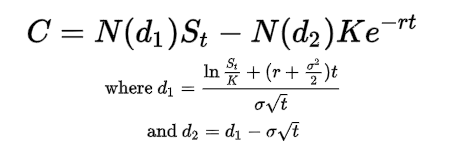# Black Scholes Model in Python for Predicting Options Premiums

Black Scholes in Python by Suhail Saqan

A stock option is the right to buy or sell a stock at an agreed price and date. The two types of options used for different situations are either calls, betting a stock will increase in value , or puts, betting a stock will decrease in value (however this is not always true, discussed at the end). Each options contract represents 100 shares of that stock.

There are also two different styles for options: American and European. The calculations that will be done here are for European options.

## Black Scholes Model

The Black Scholes model is considered to be one of the best ways of determining fair prices of options. It requires five variables: the strike price of an option, the current stock price, the time to expiration, the risk-free rate, and the volatility.Black Scholes Formula

C = call option price
N = CDF of the normal distribution
St= spot price of an asset
K = strike price
r = risk-free interest rate
t = time to maturity
σ = volatility of the asset

Assumptions Made for this Calculator

• It works on European options that can only be exercised at expiration.
• No dividends paid out during the option’s life.
• No transaction and commissions costs in buying the option.
• The returns on the underlying are normally distributed.

## Black Scholes in Python

For the Black Scholes formula, we need to calculate the probability of receiving the stock at the expiration of the option as well a the risk-adjusted probability that the option will be exercised. In order to do that we need a function for calculating d1 and d2. After that we could input them into a Normal distribution cumulative distribution function using scipy which will give us the probabilities. Each will require 5 inputs: S, K, T, r, and sigma.

Now we could implement the Black Scholes formula in Python using the values calculated from the above functions. However, there has to be two different formulas — one for the calls, and the other for puts.

## Collecting the Data

For us to test the formula on a stock we need to get the historical data for that specific stock and the other inputs related to the stock. We will be using Yahoo Finance and the pandas library to get this data.

We are able to calculate the sigma value, volatility of the stock, by multiplying the standard deviation of the stock returns over the past year by the square root of 252 (number of days the market is open over a year). As for the current price I will use the last close price in our dataset. I will also input r, the risk-free rate, as the 10-year U.S. treasury yield which you could get from ^TNX

## Utilizing the Function

We could now output the price of the option by using the function created before. For this example, I used a call option on ‘SPY’ with expiry ‘12–18–2020’ and a strike price of ‘370’. If all is done correctly we should get a value of approximately 16.169.Our Option Premium

## Implied Volatility and the Greeks

Some useful numbers to look at when dealing with options is the Implied Volatility. It is defined as the expected future volatility of the stock over the life of the option. It is directly influenced by the supply and demand of the underlying option and the market’s expectation of the stock price’s direction. It could be calculated by solving the Black Scholes equation backwards for the volatility starting with the option trading price.

Other useful numbers people look at when dealing with options are the Greeks. The following are the main five:

Delta: the sensitivity of an option’s price changes relative to the changes in the underlying asset’s price.
Gamma:
the delta’s change relative to the changes in the price of the underlying asset.
Vega:
the sensitivity of an option price relative to the volatility of the underlying asset.
Theta:
the sensitivity of the option price relative to the option’s time to maturity.
Rho:
the sensitivity of the option price relative to interest rates.

Options Pricing

I mentioned above that options prices are mainly affected by the prices however it is also important to know other factors that are used in their pricing. The three main shifting parameters are: the price of the underlying security, the time and the volatility. The implied volatility is key when measuring whether options prices are cheap or expensive. It allows traders to determine what they think the future volatility is likely to be. It is recommended to buy when the implied volatility is at its lowest as that generally means that their prices are discounted. This is because options that have high levels of implied volatility will result in high-priced option premiums. On the opposite side, when implied volatility is low, this means that the market’s expectations and demand for the option is decreasing, therefore causing prices to decrease.

## The Startup

Get smarter at building your thing. Join The Startup’s +800K followers.

## The Startup

Get smarter at building your thing. Follow to join The Startup’s +8 million monthly readers & +800K followers.## The Startup

Get smarter at building your thing. Follow to join The Startup’s +8 million monthly readers & +800K followers.

## More From Medium

Medium is an open platform where 170 million readers come to find insightful and dynamic thinking. Here, expert and undiscovered voices alike dive into the heart of any topic and bring new ideas to the surface. Learn more

Follow the writers, publications, and topics that matter to you, and you’ll see them on your homepage and in your inbox. Explore

If you have a story to tell, knowledge to share, or a perspective to offer — welcome home. It’s easy and free to post your thinking on any topic. Write on Medium